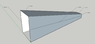# Help needed with calculating the airspeed / pressure in a funnel

• Dennis070
In summary, the airspeed at the end of the funnel can be found by using the continuity equation, but there is a caveat that the airspeed at the inlet may not be equal to the wind speed.f

#### Dennis070

Homework Statement:: Calculating airspeed and pressure in a funnel
Relevant Equations:: ?

Hi all,

I hope I posted this in the right place (if not, please let me know).

For an art project in school I want to build a funnel to direct air into a set of organ pipes. However, as mentioned, I am in art school and could really use some help.

As seen in the picture below, air (wind - let's say 10m/s) is moving in at the front. Now I need to find out (depending on the measurements of the funnel) what the airspeed is at the end of the funnel, and (in case the funnel was closed at the end) how to calculate the air pressure in Pa.

Can I use the 'equation of continuity' to calculate airspeed from A to B?

Hope someone can point me in the right direction here!

All the best,

Dennis Nijboer

#### Attachments

•Screenshot 2021-01-14 at 15.26.25.png
8.8 KB · Views: 140
Last edited by a moderator:
As long as the flow remains incompressible (in this case, slower than Mach 0.3), then simply using the continuity equation for conservation of mass will get you the answer you need.

Thanks boney3ad! I though the continuity equation only applied on liquids.

Is there a way to calculate pressure (Pa) in this situation?

Cheers!

Conservation of mass always applies, whether it's a solid, liquid, gas, plasma... At issue is just the form of the equation(s) and how many variables you have to solve. If you have a compressible flow, you can't ignore density and so you need additional equations. If it's incompressible, you're fine.

Great! Much appreciated :)

For incompressible flow, continuity equation alone will give you the velocity. To get pressure, you need to use Bernoulli's equation; however, there are two versions of the Bernoulli equation. There's an incompressible version you typically use for liquid flows and there's a compressible version. The reason I bring this up is that these two equations don't agree in the case of low Mach number gas flow. They only agree for fluids with infinitely high heat capacity ratio.
Long story short, use this version of Bernoulli's equation: $$\frac{v^2}{2} + \frac{\gamma}{\gamma - 1} \frac{p}{\rho} = constant$$ where ##\gamma = 1.4##, ##\rho## is the air density, ##p## is the air pressure, and ##v## is the flow speed. The density you can get from the atmospheric pressure and temperature using the ideal gas law. I hope that helps! Sorry for all the jargon.

Hey Twigg!

Thats all I needed to start calculating :)

Thanks to both of you!

Cheers

•berkeman
As seen in the picture below, air (wind - let's say 10m/s) is moving in at the front. Now I need to find out (depending on the measurements of the funnel) what the airspeed is at the end of the funnel, and (in case the funnel was closed at the end) how to calculate the air pressure in Pa.

Can I use the 'equation of continuity' to calculate airspeed from A to B?
There's an important caveat here, that was recently discussed in another thread: the airspeed at the inlet is likely not going to be equal to wind speed:
https://www.physicsforums.com/threa...a-funnel-be-higher-than-in-freestream.997884/

So if you already know the speed at the inlet you are fine, but if you are assuming it is equal to wind speed, you are not.

•Dennis070